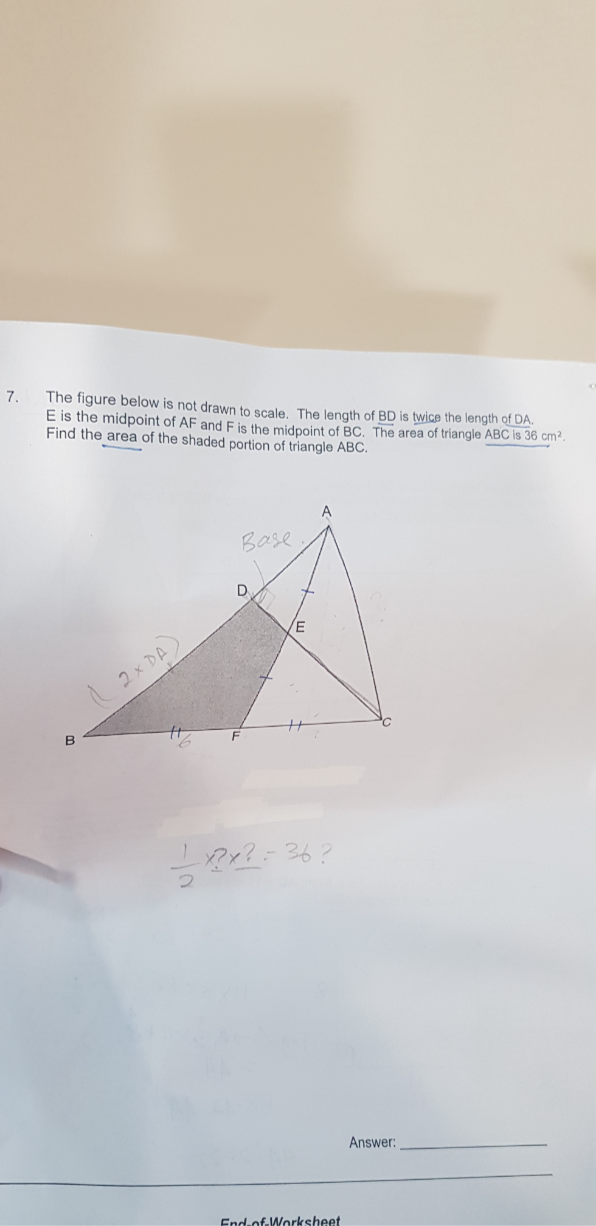# QuestionTIA!

By considering AB as base of triangle  ABC, since 2*AD = DB or AD =1/3*AB,  and the height is the same

Area of triangle ADC = 1/3 *36 = 12

By considering BC as base of triangle ABC , since BF=FC, and the height is the same

Area of triangle FCA = ½* 36= 18

By considering AF as base of triangle AFC , since FE = AE and the height is the same

Area of triangle FEC = ½*18=9